Download This Notebook: Plotting.ipynb

# Plotting

## Introduction

This tutorial describes skrf’s plotting features. If you would like to use skrf’s matplotlib interface with skrf styling, start with this

:

%matplotlib inline

:

import skrf as rf


## Plotting Methods

Plotting functions are implemented as methods of the Network class.

• Network.plot_s_re

• Network.plot_s_im

• Network.plot_s_mag

• Network.plot_s_db

Similar methods exist for Impedance (Network.z) and Admittance Parameters (Network.y),

• Network.plot_z_re

• Network.plot_z_im

• Network.plot_y_re

• Network.plot_y_im

## Smith Chart

As a first example, load a Network and plot all four s-parameters on the Smith chart.

:

from skrf import Network

ring_slot = Network('data/ring slot.s2p')
ring_slot.plot_s_smith()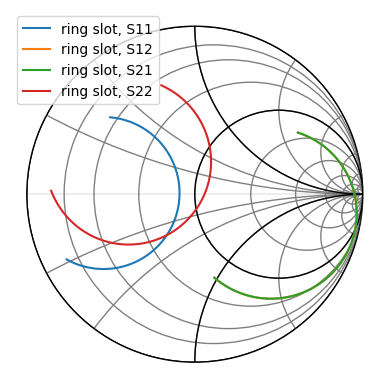scikit-rf includes a convenient command to make nicer figures quick:

:

rf.stylely()  # nicer looking. Can be configured with different styles
ring_slot.plot_s_smith()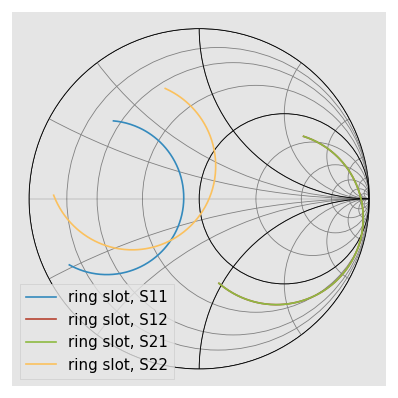:

ring_slot.plot_s_smith(draw_labels=True)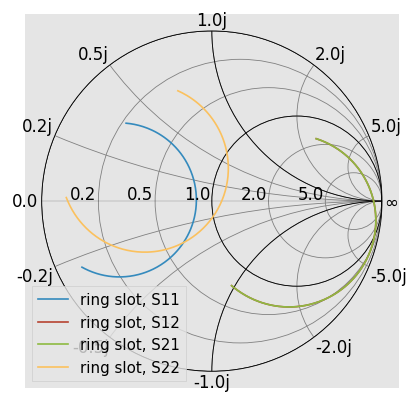Another common option is to draw admittance contours, instead of impedance. This is controlled through the chart_type argument.

:

ring_slot.plot_s_smith(chart_type='y')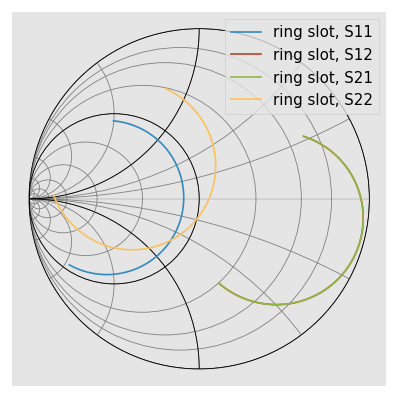See skrf.plotting.smith() for more info on customizing the Smith Chart.

## Complex Plane

Network parameters can also be plotted in the complex plane without a Smith Chart through Network.plot_s_complex.

:

ring_slot.plot_s_complex()

from matplotlib import pyplot as plt
plt.axis('equal') # otherwise circles wont be circles

:

(-0.855165798772, 0.963732138327, -0.8760764348472001, 0.9024032182652001)## Log-Magnitude

Scalar components of the complex network parameters can be plotted vs frequency as well. To plot the log-magnitude of the s-parameters vs. frequency,

:

ring_slot.plot_s_db()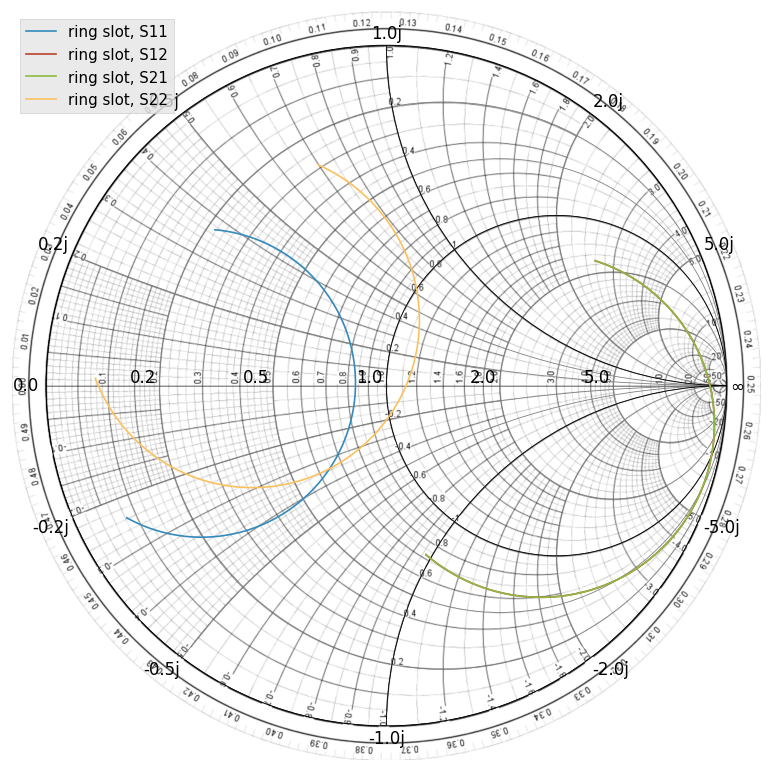When no arguments are passed to the plotting methods, all parameters are plotted. Single parameters can be plotted by passing indices m and n to the plotting commands (indexing start from 0). Comparing the simulated reflection coefficient off the ring slot to a measurement,

:

from skrf.data import ring_slot_meas
ring_slot.plot_s_db(m=0,n=0, label='Theory')
ring_slot_meas.plot_s_db(m=0,n=0, label='Measurement')## Phase

Plot phase,

:

ring_slot.plot_s_deg()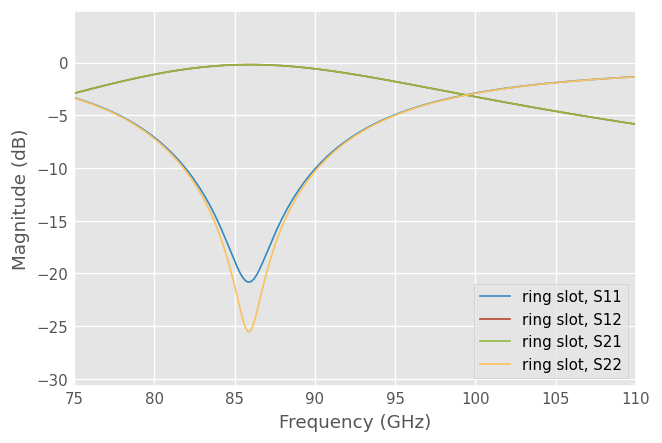Or unwrapped phase,

:

ring_slot.plot_s_deg_unwrap()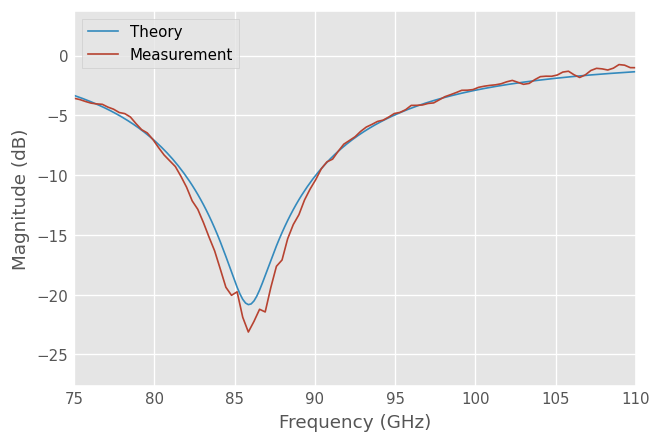## Group Delay

A Network has a plot() method which creates a rectangular plot of the argument vs frequency. This can be used to make plots are arent ‘canned’. For example group delay

:

gd = abs(ring_slot.s21.group_delay) *1e9 # in ns

ring_slot.plot(gd)
plt.ylabel('Group Delay (ns)')
plt.title('Group Delay of Ring Slot S21')

:

Text(0.5, 1.0, 'Group Delay of Ring Slot S21')The components the Impedance and Admittance parameters can be plotted similarly,

:

ring_slot.plot_z_im():

ring_slot.plot_y_im()## Customizing Plots

The legend entries are automatically filled in with the Network’s Network.name. The entry can be overridden by passing the label argument to the plot method.

:

ring_slot.plot_s_db(m=0,n=0, label = 'Simulation')The frequency unit used on the x-axis is automatically filled in from the Networks Network.frequency.unit attribute. To change the label, change the frequency’s unit.

:

ring_slot.frequency.unit = 'mhz'
ring_slot.plot_s_db(0,0)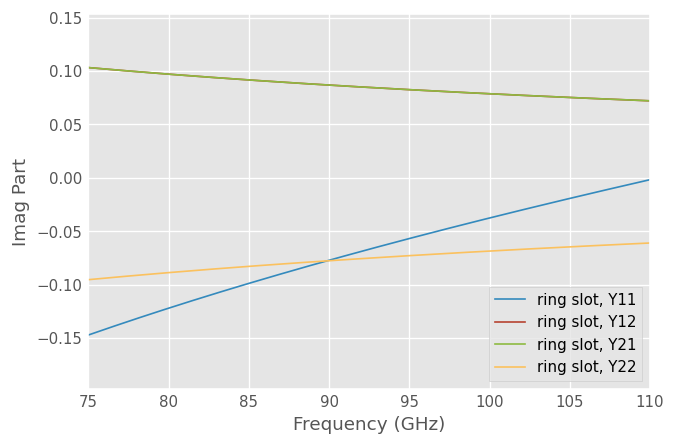Other key word arguments given to the plotting methods are passed through to the matplotlib matplotlib.pyplot.plot function.

:

ring_slot.frequency.unit='ghz'
ring_slot.plot_s_db(m=0,n=0, linewidth = 3, linestyle = '--', label = 'Simulation')
ring_slot_meas.plot_s_db(m=0,n=0, marker = 'o', markevery = 10,label = 'Measured')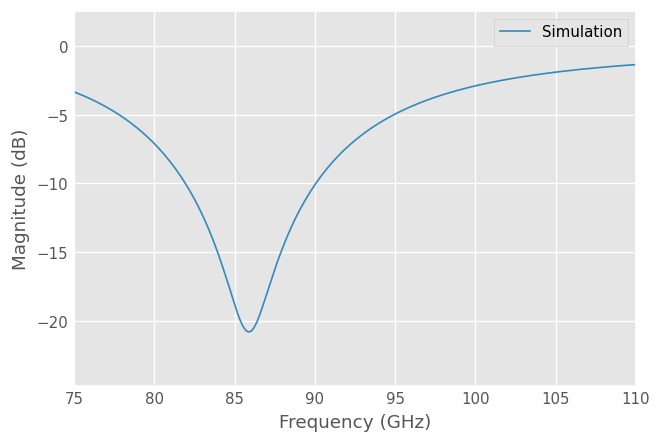All components of the plots can be customized through matplotlib functions, and styles can be used with a context manager.

:

from matplotlib import pyplot as plt
from matplotlib import style

mpl_style = "seaborn-ticks"
mpl_style = mpl_style if mpl_style in style.available else "seaborn-v0_8-ticks"

with style.context(mpl_style):
ring_slot.plot_s_smith()
plt.xlabel('Real Part')
plt.ylabel('Imaginary Part')
plt.title('Smith Chart With Legend Room')
plt.axis([-1.1,2.1,-1.1,1.1])
plt.legend(loc=5)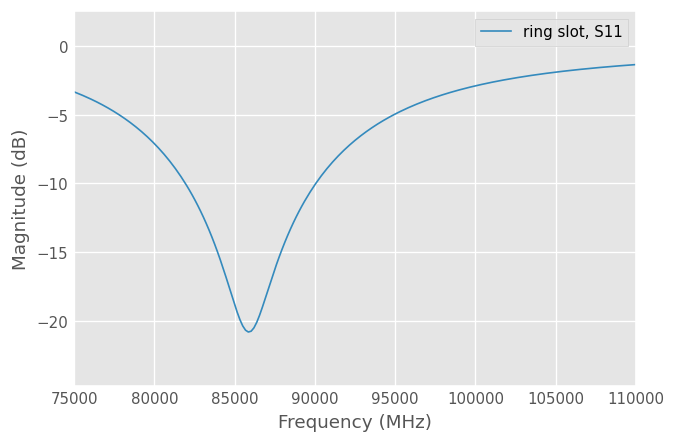## Saving Plots

Plots can be saved in various file formats using the GUI provided by the matplotlib. However, skrf provides a convenience function, called skrf.plotting.save_all_figs, that allows all open figures to be saved to disk in multiple file formats, with filenames pulled from each figure’s title,

:

from skrf.plotting import save_all_figs
save_all_figs('data/', format=['png','eps','pdf'])


A common need is to make a color plot, interpretable in greyscale print. The skrf.plotting.add_markers_to_lines adds different markers each line in a plots after the plot has been made, which is usually when you remember to add them.

:

from skrf import plotting
with plt.style.context('grayscale'):
ring_slot.plot_s_deg()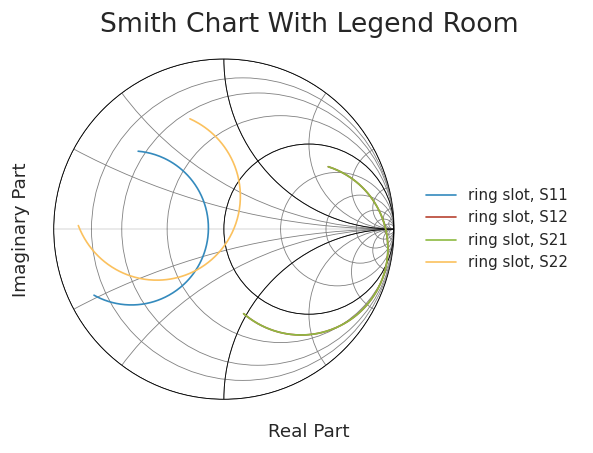[ ]: